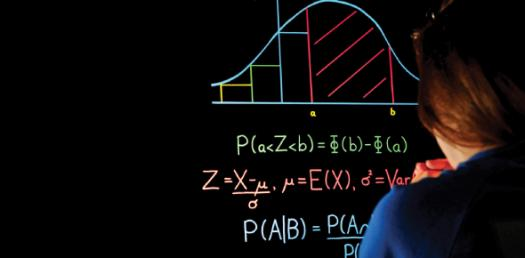# Mathematics Test: Statistics And Probability

15 Questions | Total Attempts: 321SettingsStatistics and Probability are two of the most confusing topics to people and it is important for students to get as much practice as they can to perfect their ability to tackle any questions about the two topics. The quiz below is one way in which you can do just that. Do give it a try and keep on practicing!

• 1.
It is a numeric value on an interval or ratio scale. Whole numbers that cannot be fractioned or divided. Age, number of houses, vehicles, scores of the game, etc.
• A.

None of these

• B.

Nominal

• C.

Ordinal

• D.

Discrete or Scale Data

• 2.
Data values represent categories with some intrinsic order or property of magnitude, e.g., vote yes, no or conditional; agree, partially agree, disagree, Letter Grades ABCDEF, etc.
• A.

Ordinal

• B.

Nominal

• C.

Discrete or Scale Data

• D.

Continous

• 3.
Data values represent categories with no intrinsic order e.g. male/female, Tagalog/Visayan/Lumad, blood type, color of hair, parcel numbers, ID numbers, license plate number, etc.
• A.

Discrete or Scale Data

• B.

Nominal

• C.

Ordinal

• D.

Unmeasurable

• 4.
Sum of items divided by the count of items.
• A.

None of these

• B.

Median

• C.

Mode

• D.

Mean

• 5.
Value of a set of observations which occur most often or with the greatest frequency; Data sets can have more than mode, while the mean and median have one unique value.
• A.

Mode

• B.

Mean

• C.

Median

• D.

Value

• 6.
Denoting or relating to a value or quantity lying at the midpoint of a frequency distribution of observed values or quantities, such that there is an equal probability of falling above or below it.
• A.

Mean

• B.

Mode

• C.

Median

• D.

None of thess

• 7.
Is a list of all the individuals (units) in the population from which the sample is taken.
• A.

Convenience sampling

• B.

Purposive sampling

• C.

Quota sampling

• D.

Sampling frame

• 8.
Number units within the sampling frame and select 5th, 10th, etc.
• A.

Stratified Random

• B.

Systematic Random

• C.

Simple Random

• D.

Multi-stage Random

• 9.
Selection on the basis of categories that are assumed to exist within a population.
• A.

Sampling frame

• B.

Cluster

• C.

Quota sampling

• D.

Purposive sampling

• E.

Convenience sampling

• 10.
Is a systematic approach to estimating the strengths and weaknesses of alternatives that satisfy transactions, activities or functional requirements for a business, sometimes called BCA.
• A.

Cost effectiveness analysis

• B.

Cost estimate analysis

• C.

Cost revenue analysis

• D.

Cost benefit analysis

• 11.
Tools of planning research which is an indirect measure of a present or past condition that can not be directly measured because of lack of clear data, lack of time, or cost to do the full study.
• A.

Estimate

• B.

Projection

• C.

Forecast

• D.

Targets

• 12.
Tools of planning research which is a conditional statement about the future. Estimates of future populations based on statistical models that extrapolate past and present trends into the future.
• A.

Estimate

• B.

Projection

• C.

Forecast

• D.

Targets

• 13.
Tools of planning research which is a judgmental statement of what the analyst believes to the most likely future.
• A.

Estimate

• B.

Projection

• C.

Forecast

• D.

Targets

• 14.
In all distributions of data, the median is equivalent to the:
• A.

Mode

• B.

Variance

• C.

50th percentile

• D.

Mean

• 15.
This refers to the broad type of social research whose central objective is to solve urgent problems, develop a tool or social technology, explain effects of policies or actions and evaluate the impact of plan and programs on people.
• A.

Exploratory Research

• B.

Pure/Basic Research

• C.

Evaluation Research

• D.

Applied Research

Related TopicsBack to top